Gravimotion Interpretation of Reality

Motion energy. The kinetic energy of any object in constant linear motion maintains it in constant linear motion unless acted upon (Newton's inertia law); and any light wave flow of energy does so at constant speed.
In this gravimotion interpretation, an object's kinetic energy and light's flow of energy are of same nature represented as a motion-volume.

The motion-volume. Light rays are occurring as photons, which while massless can be observed and measured, as such a photon occupies a volume of space-time, given the name motion-volume here.
A motion-volume implements both the photon's energy and its speed. A Motion-volume represents a Real entity, breaking with science's motion of material objects.
Regarding its volume, unlike an object's volume a motion-volume is massless and its three dimensions involve lengths in space but also a time duration.
Just as a material object's volume though, it's time duration is finite, it is lasting the time duration of the present, as nothing exists in the past and nothing exists in the future; yet a motion-volume renews itself over and over at present, just as any material object has the ability to do; a uniform motion-volume continues unaffected unless modified by collision or overlap by another motion-volume.
In order to comply with our human concept of energy, the duration in time of a motion-volume is the inverse of a conventional time duration; it is expressed in inverse seconds; now the longer the duration of a motion-volume in inverse seconds the higher its motion or moving energy.In this animation, the motion-volume is a cylinder, which volume is the product of its cross section (the circle's surface in space) by a time duration, that is the time represented by the bottom line in inverse seconds.
The motion-volume's fabric, that is motion (one word), reflects the fact that time and space (two words) make one, a fact first discovered and mentioned by Einstein.
Using the Standard Unit of Measurement, the unit of a motion-volume is 1 inverse seconds . 1 meter2.
Beware, neither motion's reality nor this mathematical representation are considered in science physics.

Introduction. In gravimotion interpretation, motion is an integral part of the present moment that is the reality occurring as space time and all that is within. That implies, as shown next, that space-time is occurring under motion-volumes; and, also that particles matter popping out of this sea of motion-volumes (space time) are occurring under motion-volumes concatenations.
Please note, considering that Reality whether non material or material is occurring under motions, that have finite durations while renewing themselves, is departing from science's Standard Model in which everything is made of particles.
Besides should you decide to read about gravimotion, this Frenchman's english writing is not the best.

Space time in science. Michelson and Morley in 1887 made an experiment proving beyond any doubt there is no "aether" in space. Yet in 1915 Einstein's general relativity describes how gravity bends and stretches space-time, proving instead spacetime must have a physical fabric.
Dark energy, which is causing the Universe's expansion, big bang and the cosmic microwave background, are all involving some type of motion, yet none of these provide a clue regarding the fabric of spacetime.
And popping out a quantum field, are particles / antiparticles whose pairs amount to nothing, again justifying the emptiness of space-time while incomprehensibly contradicting the existence of such quantum fields; in addition the 2009 optical resonator experiments confirmed the absence of any aether.
Here, this "aether" emptiness is challenged, spacetime must have a volume just as the Universe has; next spacetime is provided a physical fabric actually turning out to be an active entity.

Spacetime is given the name present mo(ve)ment. Space-time is a Reality that occupies a volume, as if space-time had no volume our Sun would be in contact with our Earth atmosphere; you and I would not exist. Here, the entity space-time is determined by analogy to the temperature of the air surrounding us.
• Maxwell showed how the air temperature is coincidental to the air molecules' kinetic energy, which is interpreted here as motion-volumes animating the air molecules. Sound waves (voice, music) are tuned vibrations of these motion-volumes activity.
• Because light waves are oscillations occurring within space time, one is entitled to think that light waves are occurring as motion-volumes, which are tuned vibrations of a background made of motion-volumes at the image of the air molecules activity.
Represented here is the motion of the air molecules surrounding you; the molecules have been removed, their motion only shows.
This motion is also the image of space time.
•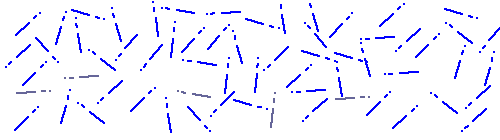Space-time is then considered here to be occurring under haphazardly oriented motion-volumes.
To distinguish them from light waves, these motion-volumes are labelled present mo(ve)ment, unveiling space-time (the present moment) Reality.
• The present mo(ve)ment is occurring as massless motions occurring in all directions, ephemeral along the present, yet indefinitely renewing itself throughout the entire Universe that is throughout space-time.
• Because this present mo(ve)ment allows for light-speed, its motion-volumes are moving at light-speed.
• Getting back to above analogy, in reverse, just as light speed is constant in the present movement, sound speed is constant in constant temperature air.
Being massless and having no specific direction the present mo(ve)ment activity is temperature less, invisible and impalpable; when measured, the thermometer used remains at its minimum.
Renewing itself over and over at present throughout the entire Universe, besides being self-driven that is active, the present mo(ve)ment is altogether finite (the duration of the present) and infinite (the present renews itself over and over).

Particle matter and surrounding gravity. Take your pencil, lift it and release it. Your pencil is getting into motion, falling to the ground. Gravity, a Reality called gravitational acceleration in science, is occurring as motion hence the word gravimotion,.
And the sensation we feel under our feet while standing up amounts to a stalled motion directed toward a center of gravity. Gravity is occurring under invisible stalled motion-volumes.
In gravimotion's interpretation, a particle matter is occurring at the image of gravity that is accelerated motions all directed toward the same center of gravity yet at a much higher density than gravity; gravity being now a byproduct of the matter it is surrounding.
At the origin of any material particle, 2 motion-volumes, within the present mo(ve)ment, collide head on and remain frozen against each other, attracting a number of other pairs around their collision center.
Both matter and surrounding gravity are organized alterations of the present mo(ve)ment; both occur as motion-volumes all directed toward a common center of gravity, yet stalled around it, in effect implementing our human concept of inertia.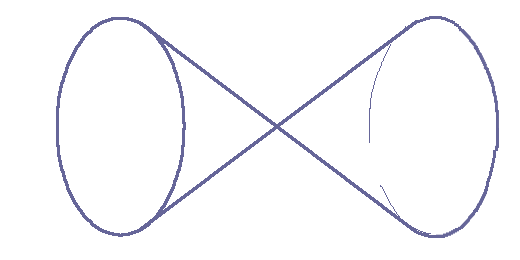When a head-on collision occurs, as shown left, both motion-volumes get compacted as shown right; their time durations get shorter and shorter toward the center, expressed mathematically as a squared time; each motion-volume becoming an accelerated motion written inverse seconds2 . meter2. Such acceleration brings to mind the C2 appearing in Einstein's famous equation E = m C2.
In this process, some of the present mo(ve)ment (space-time) in which this interaction is occurring is pushed-out, and by reaction is oriented in the opposite direction toward the center establishing the gravimotion surrounding it.In effect this nucleus creates in its surrounding a frozen acceleration of the present, amounting to the gravity surrounding any material entity.
This provides the physical mechanism acting behind matter / gravity inter dependency. The left illustration slows down at the end of each cycle to allow observation of the gravimotion surrounding the newly created particle.
Our human concepts of particle matter and gravity are, in gravimotion, integral part of the present mo(ve)ment; yet particle matter motion-volume pairs are compounded in such high density they cannot be penetrated by other particles of similar constitution.
The present mo(ve)ment's motion-volumes in blue are invisible to human eyes; the material subatomic particle popping out of it is represented in black.
In quantum mechanics, as already mentioned, particles made of energy pop haphazardly out of empty space; and to justify this emptiness (science's space-time nonexistence), an antiparticle is popping out each time a particle does; in gravimotion, space-time is not empty, it is instead occurring as motion-volumes out of which pop out particles configured as motion-volumes.
There is neither a creation nor a loss of energy; material particles are only harnessing the present or modifying it.

The particule's matter numerical expression. Science's kinetic energy is E = 1/2 m . v2, the factor m being the particle's mass and v2 its speed squared.
• In gravimotion the expression kinetic energy takes its full meaning, and is considered to be the fabric of any material particle. The mathematical factor v2 takes over and becomes the major actor at the expense of the factor m.
• the factor m is devalued to a pure number, a quantity having no unit; mathematically the squared velocity takes over science's inertia momentum.
Considering now Einstein famous equation E = m . C2, a particle matter is occurring as a number (# for m) of light speed squared, written here # . meter2/second2.
And in terms of inversed time, a particle matter numerical expression is: # . inverse-second2 . 1 meter2.
A particle matter is implemented as a “number” of mass-less motion-volumes, which happen to fit the photon's numerical expression.
Note that while mass m takes precedence over light speed C regarding the constitution of a particle matter in science, some scientists wonder what mass is made of.

Motion of Particle matter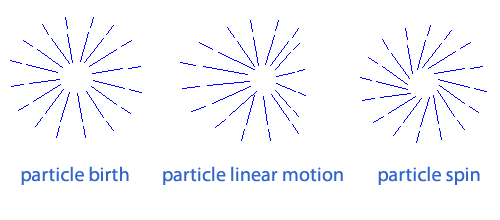An object is in motion anytime the motion-volumes pairs constituting it are set out of equilibrium; such an out of equilibrium state is provided by external motion entering it or overlapping it and creating within it a loss of equilibrium; this in accordance with science's principle of “energy” conservation.
The illustration shows only the modification of the gravimotion surrounding the matter; an identical modification occurs within matter itself; setting out of equilibrium the center of gravity, which in science is used to define the motion of the particle.
A spin constant motion just as a linear uniform motion does not require any additional incurring motion to occur; even though not mentioned in it, constant spin is part of Newton's first law. Turns, on the other hand, require out of line motion to interact. Gravimotion's (the gravity surrounding mass) modifications into linear and spinning motions are not part of science.

Introducing swirling volumes The hand of the clock on the right side is rotating clockwise (CW).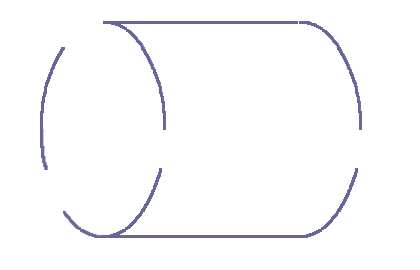And the motion-volume shown on its back exhibits an identical CW swirling; the latter is referred to as a CW volume in the following.
On the left a counter clockwise swirling volume referred to as a CCW volume is shown.
Now just as gravimotion's motion-volumes, these swirling volumes are not part of science and do not exist in science.
Please note that looking at the back of those 2 swirling volumes, the CW volume is seen as rotating CCW and the CCW as a CW; no matter our human thinking, these two are spinning in opposite directions when both are observed from the left.
Swirling and spinning refer to rotations, in the following the words swirling, spinning, circling, rotating, curling may be used.

Electrical Particles' fabric. In science physics there are positive and negative electric particles, which are made of quarks that are counted in thirds of electric charges; yet these electric charges are provided with no physical fabric other than asserting they are charges that bear positive or negative mathematical sign. The goal here is to unravel the physical structure occurring behind these so-called positive and negative charges.
1. As mentioned in "Particle matter and surrounding gravity" section, a material particle is occurring as a bunch of motion-volume pairs; the 2 motion-volumes of each pair butting at each other at a center of gravity.
2. In science electric particles of opposite sign attract each other; on the other hand two positive charges repel each other, as do two negative charges.
3. Matter's motion-volume pairs (item 1) now made swirling do implement the physical structure of an electric charge as defined in science (item 2); a positive charge is physically implemented by CW volumes pairs, a negative charge by CCW volumes pairs, as described next.
Within the present mo(ve)ment (space time), the collision of 2 motion-volumes rushing against each other is ending in an inert stall creating a particle matter; yet the collided activity may in addition be stored under spinning motions, in such a way the particle is balanced, the process still complying with energy conservation.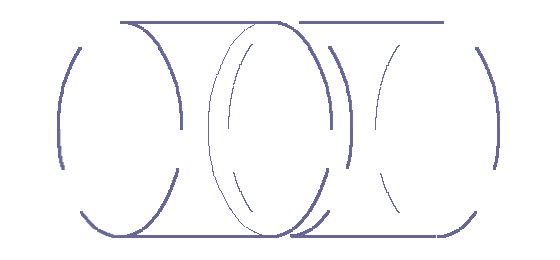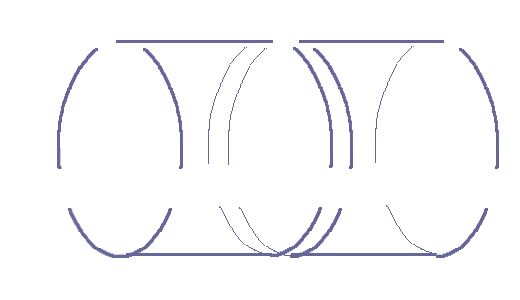As shown on the left, for an electrically negative particle such as an electron, the pairs collided on their respective CW front ends; now the spinning as viewed from either end of the pairs are circling CCW; the 2 motion-volumes find themselves entwined at the center into a strong CW CCW embrace, along science's electric positive negative strong attraction; and as shown right, both ends of a positive charge are observed spinning CW.
Just as in science in which the closer to the center of an electric charge the stronger the field, here the closer to the center of the rotating activity the higher its density and intensity;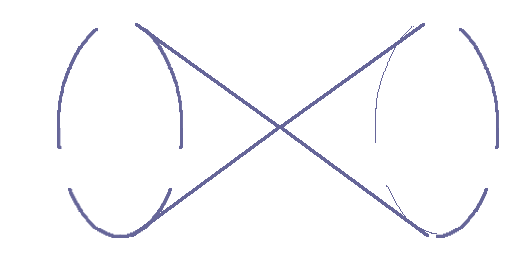the farther from the center the weaker this rotating activity. This is represented at right in which the closer the swirling to the center the greater the activity per unit volume. With respect to the center, 2 symmetrical volumes are spinning in opposite directions, which is keeping the pair balanced unless modified by appropriate external motion.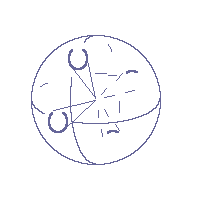A CW particle (positive particle ) is the assembly of a number of pairs; in actuality the particle's entire volume is filled with pairs; the individual pairs' volume restriction at center allow the whole particle final volume; on the left is the schematic of a CW particle in which only 2 CW pairs are shown.
CW and CCW volumes are, in gravimotion, the physical structures implementing science particle's positive and negative mathematical signs.
Just as a particle matter is modifying its surrounding present mo(ve)mentn into gravimotion, an electrical particle spinning activity is modifying its surrounding present into spinning activity.

Electric field. Mutual interactions between the present and electrical CW and CCW particles are inducing CW and CCW curlings in this very present surrounding them, physically implementing science's electric fields.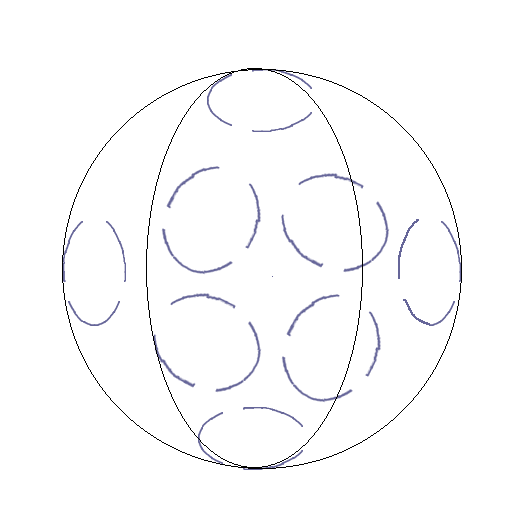The visible half sphere on the left is representing part of the present mo(ve)ment (space-time) surrounding the above positive particle; it displays 8 spinning CW volumes corresponding to 8 CW pairs; yet unlike above their opposite symmetrical corresponding 8 CW volumes are not represented.
The present (space time) surrounding the particle is curled following the very particle's swirlings, its intensity is decreasing with distance in accordance to the increase of the circling volumes cross sections constituting them.
And just as science's positive charges are behaving, the clockwise-curlings surrounding CW particles are repulsing other CW particles surroundings; on the other hand CW and CCW particles surroundings work like hand in glove.
Gravimotion (a particle's surrounding gravity) remains oriented toward the center of gravity, whether the particle surroundings exhibit electrical curlings or not.

Electrical conductor Matter is composed of atoms each made of a nucleus around which are circling CCW particles of type electrons; the outer circling electrons are called here peripheral electrons riding peripheral trajectories; in metals such as copper these peripheral trajectories are oriented in all directions and the peripheral electrons are free to jump between the atoms peripheral trajectories; when prompted by an external electrical activity all these electrons move in orderly direction within the wire along the orientation of the activity (along time); copper is a conductor; per definition a conductor offers free passage to a flow of electrons called electric current.
Within a battery, a number of CW particles are kept away (forming the positive pole) from a number of CCW particles (the battery negative pole); viewed from the outside that amounts to 2 stalled activities (science's positive and negative electric field), which when connected through an external conductor wire of electricity engenders an electric current.

Magnetic activity surrounding any electrical current. In science physics any electrical current, that is a number of electrons moving in a unified motion, implements in its surrounding a magnetic field. The right hand rule provides the direction of the magnetic field. Set your right hand along the wire carrying a current of electrons, your right thumb in the direction of the current, the wrapping of your other fingers are in the direction of the magnetic field.
In gravimotion the surrounding of the wire that is space-time called the present mo(ve)ment is occurring as a sea of uninhibited motions; the current in the wire modifies this surrounding present motions into circling motions round the wire.

Magnets. Iron and nickel are conductors that can be turned into magnets. Placing an iron rod within a strong CW (North) CCW (South) magnetic activity modifies its atoms' peripheral trajectories.The external CW CCW present mo(ve)ment revolutions penetrate the rod and have the effect to orient the atom's peripheral trajectories parallel to each other as shown on the left and the peripheral electrons are forced to be circling in concert.
Once the external strong North South magnetic activity has been removed, the peripheral electrons continue circling in tune with each other, which keeps their peripheral trajectories aligned, the whole amounting to a circling electrical current enforcing the rod's own magnetic activity. CW and CCW circlings on ends are enforcing the North and South poles of the new magnet,
This magnet in turn is implementing circular motions in its surroundings (science's magnetic field).
At the image of gravitational matter which is re-orienting the present (space-time) surrounding it, magnets are re-orienting the very motions of the present mo(ve)ment surrounding them yet in a different way.

Electromagnetic waves and photons In the following in order to simplify the language, only a small spectrum of the electromagnetic waves that is light waves and their photons are considered.

The photon's numerical expression In science a photon is energy provided by Planck's equation E = h . f .
h is Planck's constant and f the frequency characterising the photon considered; a frequency being defined as a "number of cycles per second".
• In gravimotion, a photon cycle (the very word used in "number of cycles per second") is the
period
A cycle could as well be chosen to be a wavelength, as in De Broglie relation, which involves momentum instead of energy; in that case the number of cycles per second becomes a speed.
This word cycle as used in science, actually ends up being either a period (a time duration) or a wavelength (a length in space), which amounts to a lack of definition; science's cycle amounts to an undefined entity, with no measuring unit, rendering the Hertz (so called unit) abstract that is non phyical.
of the wave defined by the frequency f and expressed in inverse seconds; for instance, a photon of frequency f = 10 periods per second, is a photon whose period cycle is occurring in .1 second. f = 1 / period duration in seconds.
Mathematically f is the inverse of a time period and E = h . inverse-seconds.
• Planck's constant is h = kg . 6 10-34 meter2 / second; in gravimotion interpretation the kg is a number just as 6 10-34 is.
Planck's constant becomes # . meter2 / second or # . inverse-second . 1 meter2
Considering f inverse time, a photon is occurring as a # . inverse-seconds2 . 1 meter2, which is also the particle matter mumerical expression in this gravimotion interpretation.
In gravimotion interpretation of Nature, a photon so called particle is occurring as a motion-volume coinciding to a single cycle of a wave, and is behaving just as the wave does.
Or it could be said that any light wave is acting as a succession of motion-volumes (particle photons) each coinciding to a cycle of the wave.
Occurring over a time duration equal to its time period, science's particle photon is integral part of a wave and that abolishes light's particle/wave duality dilemma.

The photons' inner mechanism. Beneath these succint mathematics, is an intricate inner mechanism.
In this animation, single photons are crossing your screen's surface, popping out of it one after another; each is occurring as a motion-volume defined as a cylinder having for base the surface area of the top circle and for length a duration coinciding to the the full revolution of the blue radius, precisely lasting the inverse of the wave's period.
Beware, while based on science physics, a photons' activity or motion-volume departs greatly from science's particle photon. In science the photon's frequency is energy; when considering the wave rather than the particle, such energy is occuring as fields graphically represented with two electric and eletromagnetic sinusoids offset 900 degrees with respect to each other; when either one of these two fields is null the other is at an absolute maximum, as such a photon can be represented as done here as two simultaneous sine and cosine events.
The animation below coincides with a specific polarized light, such as a blue photon for instance, but represented at a much lower frequency.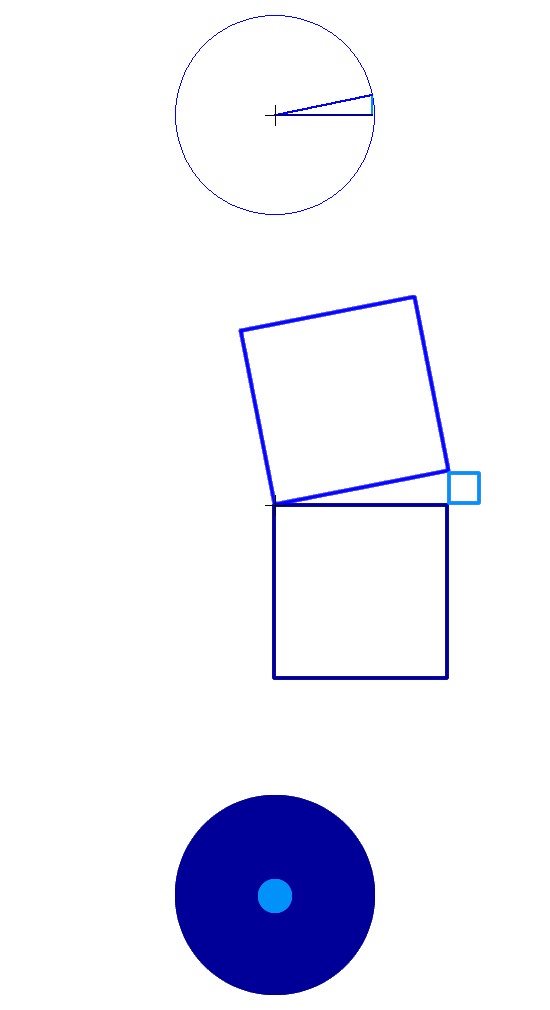In the top animation the plain blue revolving radius of length r determines successive angles; the cosines of these angles (the dark blue horizontal lines) coincide to the electric field variation; the sines (the light blue vertical lines) coincide to the magnetic field; the dark and light blue line that is the electric and magnetic fields are at 90 degrees with respect to each other just as in science physics. Usually, in mathematics, the sine is represented coincident to the vertical axle; here the sine lines are shifted to the end of the cosines.

In the second animation, squares are attached to these 3; according to Pythagorean's theorem the plain blue radius squared: r2 is at all times equal to the horizontal cosine squared plus the vertical sine squared; here the radius, cosine and sine lengths are multiplied by √ Π (read square root of pie) so the area of the squares attached to the revolving radius (in plain blue) are at all times unchanged and precisely equal to the surface of above circle; the light and dark blue squares areas only are varying. And as can be seen when either of these two squares' area is maximum the other is minimum.

In gravimotion the electric-magnetic duality occurs as follows: the electric component (dark blue squares) below the horizontal to start with, while decreasing is transferring its energy to the magnetic (light blue squares) above the horizontal, which as a consequence is increasing; when this magnetic component reaches a maximum it rebounds within the common allocated motion-volume, and is restituting back the energy to the electric component; a full cycle is involving 4 transfers.

The third animation illustrates this interaction; the dark blue surface (cosine squared or electric field) and the lightblue surface (sine squared or magnetic field) are mutually interacting with each other over a full period.

The photon's activity. In gravimotion interpretation, the present mo(ve)ment (science's space-time) is occurring at the image of the air molecules motions surrounding us out of which the material molecules have been removed and within which light waves rather than sound waves are travelling; the photon's are occurring as well defined oscillations of the present mo(ve)ment, which is illustrated in above section Spacetime is given the name present mo(ve)ment.In this illustration, rather than popping out of the screen and travelling toward your eyes, the photons are travelling left to right; it highlights the fact that when the electric field is maximum (outside perimeter dark blue) it is also moving fast in space that is browsing great distances in little time; on the other hand, when the magnetic field is maximum (outside perimeter light blue) the photon motion-volume is taking long time durations to cover short distances. In this illustration, as above, the photon's activity is greatly slowed down to highlight this intertwining.
Furthermore the back and force electric to magnetic volumes synergy is interacting with the un-coordinated motion-volumes sea (space-time) within which it is progressing, actually modifying the latter along and producing the photon's overall linear motion; at the image of your own walking, in which the energy propelling you is your very own body acting on the outside ground.
The direction of the photon's activity is remaining as is unless modified, it is determined by the emitter of the photon.

The present mo(ve)ment (spacetime) numerics. In the absence of the electromagnetic wave energy, the above plain blue radiuses rather than oscillating in harmony have erratic angular orientations implementing the present mo(ve)ment (space time).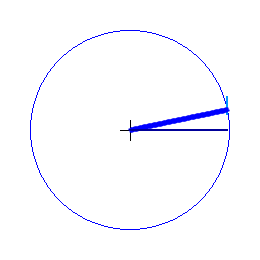The horizontal dark blue cosine line's are considered to be variations occurring in space; the vertical light blue sine line's occurring at 90 degrees are covering no space and are varying in time only.
The motion-volumes making the present are fundamental motion-volumes in which there is no cyclic oscillation and no gravity.
Planck's constant 6.62607015 x 10-34 . 1 kg . 1 meter2 / 1 second, re-written here:
66 10-33 . 1 inverse-second . 1 meter2
is the numerical value of a fundamental motion-volume.
For you and I, 10-33 is a decillionth that is 0 . 000 000 000 000 000 000 000 000 000 000 000 1 a very small space-time duration indeed.
This fundamental motion-volume is the fundamental block for space time, the electromagnetic waves and science's fundamental elements. It is fundamental as the inverse-second term is not squared as it is in matter and electromagnetic waves motion-volumes numeric interpretations.
The present is self propelling, an activity constantly renewing itself, a fact we are all witnessing at each instant of our lives, hence the appellation present mo(ve)ment.
Even though advocated by Einstein this space-time-oneness is difficult to imagine as most of us human beings think that time is running independently of anything else and more so independently of space.
The way to answer is to note that the present only is real; to morrow's world even the world of the next second does not exist; and yesterday's entire Universe along that of last second does no longer exist; the past just as the future are never in force; everything that surrounds us in space, along the whole physical Universe, is constantly renewing itself along the present.
This present constant renewing has fundamental consequences; first it keeps the identity of things alive allowing them not to disappear in between (what we call) two successive time instants; then it allows evolution; these two fundamentals in turn are mandating their cause, which is constant renewed duration.
Such short mo(ve)ments can only be occurring as motion-volume's quantums or activity quantums.

Regarding the analogy space-time / air temperature used here in the first place
Per definition inert inertia (inert mass) cannot be kinetic movement (kinetic energy) as is the particle matter in science. And to corroborate, the photon is proof that activity (moving energy) is occurring independently of inert mass.
In our human quest for unification, rather than identifying the electromagnetic wave to a particle as done in science, we may as well identify the particle matter to motion as done in gravimotion.
Now this devalues our concept of mass to a number having no unit; a situation incompatible with Einstein's equation E = mC2, kinetic energy E = mv2 and Boltzmann temperature T = m v2 / 3k; as unit wise the term E would be thrown off balance.
Yet, based on the units of measurement, the following shows a temperature T is equal to a speed squared v2.
The Calorie, an old unit of energy, has been redefined as being equivalent to 4.2 Joules. 1 calorie (thermo chemical) = 4.184 joule exactly.
1 calorie = 4.184 joule (1)
The Joule, a unit of energy equivalent to a force multiplied by distance, is defined as 1 Joule = 1 Newton x 1 meter.
1 calorie = 4.184 x 1 Newton x 1 meter (2)
The Newton, a unit of force equivalent to the acceleration of matter, is defined as 1 Newton = 1 kilogram of matter accelerated at the rate of 1 meter per second second.
1 calorie = 4.184 x 1 Kg x (1 meter/ 1 sec sec) x 1 meter (3)
or:
1 calorie = 4.184 x 1 Kg x 1 meter2 / (1 sec)2 But the calorie a unit of heat (heat being a specific form of energy), is defined as being the heat added to one gram of water to raise it from 14.5 to 15,5 degree Celsius.
1o Celsius x 1 gram = 4.184 x 1 Kg x (1 meter)2 / (1 sec)2(4)
or:
1o Celsius x 1 gram = 4.184 x 1000 gram x (1 meter)2 / (1 sec)2(5)
which can be rewritten:
1o Celsius x 1 gram = 4,184 gram x (1 meter)2 / (1 sec)2(6)
and dividing each side by one gram:
1o Celsius = 4,184 (1 meter)2 / (1 sec)2 (7)
Or within a constant :
To = v2(8)
A temperature change of 1 degree, is equivalent to a speed squared of 1 meter2 / 1 second2; and a # T of degrees is a # of 1 meter2 / 1 second2.
Written in terms of motion-volumes as # of inverse-second2 . 1 meter2.
This justifies, in this mind of mine, the assumption that space-time is occurring at the image of the air molecules movements and called the the present mo(ve)ment.

Copyright © 2022 Henri Salles. You have the permission to reproduce, print, distribute and post the contents of this website, provided you mention the link: https://gravimotion.com. Contact henriJMsalles[at]gmail.com.